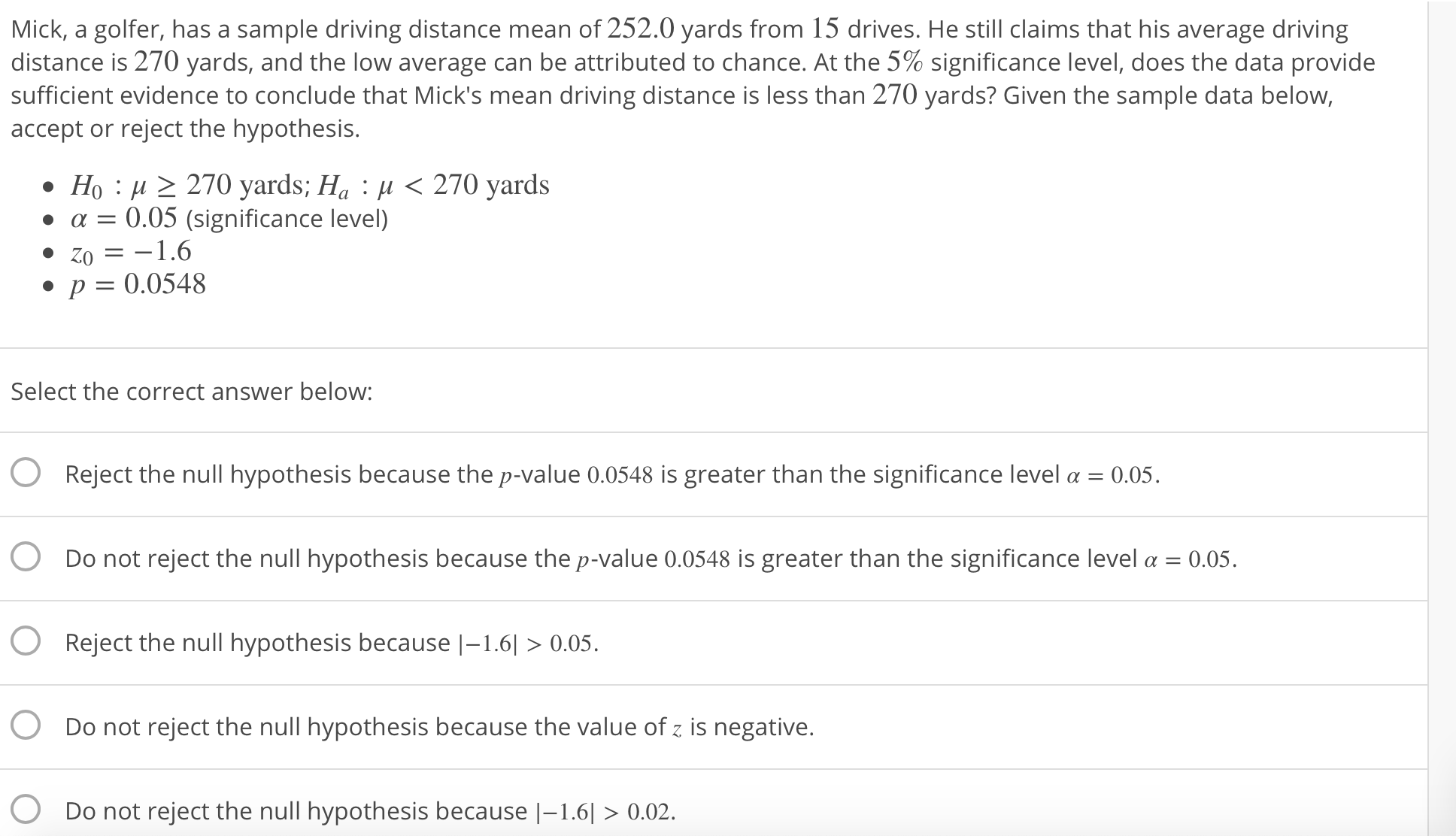# Mick, a golfer, has a sample driving distance mean of 252.0 yards from 15 drives. He still claims that his average drivingdistance is 270 yards, and the low average can be attributed to chance. At the 5% significance level, does the data providesufficient evidence to conclude that Mick's mean driving distance is less than 270 yards? Given the sample data below,accept or reject the hypothesis.Ho H2270 yards; Ha0.05.Do not reject the null hypothesis because the value of z is negative.Do not reject the null hypothesis because |-1.6>0.02.

Question
81 viewshelp_outlineImage TranscriptioncloseMick, a golfer, has a sample driving distance mean of 252.0 yards from 15 drives. He still claims that his average driving distance is 270 yards, and the low average can be attributed to chance. At the 5% significance level, does the data provide sufficient evidence to conclude that Mick's mean driving distance is less than 270 yards? Given the sample data below, accept or reject the hypothesis. Ho H2270 yards; Ha<270 yards α 0.05 (significance level) Zo=-1.6 p0.0548 Select the correct answer below: O Reject the null hypothesis because the p-value 0.0548 is greater than the significance level a 0.05. O Do not reject the null hypothesis because the p-value 0.0548 is greater than the significance level α-0.05. O Reject the null hypothesis because-1.6l>0.05. Do not reject the null hypothesis because the value of z is negative. Do not reject the null hypothesis because |-1.6>0.02. fullscreen
check_circle

star
star
star
star
star
1 Rating
Step 1

Decision based on p-value:

At level of significance α, if the p-value ≤ α, then reject the null hypothesis, H0. Otherwise fail to reject H0.

If H0 is rejected, then it can be interpreted that “there is enough evidence to suggest that  H0 is false, that is, to support the claim made by the alternate hypothesis, Ha at level of significance α”.

Step 2

Interpretation:

Here, the level of significance is α = 0.05 and p-value =0.0548 and z0= –1.6. The null hypothesis is rejected when p value is less than the level of significance. Therefore, 1st option is incorrect.

Now, p-value is 0.0548, which is greater than significance level α = 0.05. Therefore, by decision rule, do not reject the null hypothesis. Therefore, 2nd option is correct.

There is no decision rule connecting p-value and the t...

### Want to see the full answer?

See Solution

#### Want to see this answer and more?

Solutions are written by subject experts who are available 24/7. Questions are typically answered within 1 hour.*

See Solution
*Response times may vary by subject and question.
Tagged in

### Other